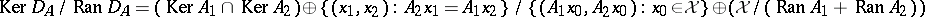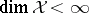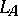# Taylor joint spectrum

Let $\Lambda \equiv \Lambda [ e ] \equiv \Lambda _ { n } [ e ]$ be the exterior algebra on $n$ generators $e _ { 1 } , \ldots , e _ { n }$, with identity $e _ { 0 } \equiv 1$. $\Lambda$ is the algebra of forms in $e _ { 1 } , \ldots , e _ { n }$ with complex coefficients, subject to the collapsing property $e _ { i } e _ { j } + e _ { j } e _ { i } = 0$ ($1 \leq i , j \leq n$). Let $E _ { i } : \Lambda \rightarrow \Lambda$ denote the creation operator, given by $E _ { i } \xi : = e _ { i } \xi$ ($\xi \in \Lambda$, $1 \leq i \leq n$). If one declares $\{ e _ { i_1 } , \ldots , e _ { i_k } , i , 1 \leq i _ { 1 } < \ldots < i _ { k } \leq n \}$ to be an orthonormal basis, the exterior algebra $\Lambda$ becomes a Hilbert space, admitting an orthogonal decomposition $\Lambda = \oplus _ { k = 1 } ^ { n } \Lambda ^ { k }$, where $\operatorname { dim } \Lambda ^ { k } = \left( \begin{array} { l } { n } \\ { k } \end{array} \right)$. Thus, each $\xi \in \Lambda$ admits a unique orthogonal decomposition $\xi = e _ { i } \xi ^ { \prime } + \xi ^ { \prime \prime }$, where $\xi ^ { \prime }$ and $\xi ''$ have no $e _ { i }$ contribution. It then readily follows that $E _ { i } ^ { * } \xi = \xi ^ { \prime }$. Indeed, each $E_i$ is a partial isometry, satisfying $E _ { i } ^ { * } E _ { j } + E _ { j } E _ { i } ^ { * } = \delta _ { i j }$ ($1 \leq i , j \leq n$).

Let $\mathcal{X}$ be a normed space, let $A \equiv ( A _ { 1 } , \dots , A _ { n } )$ be a commuting $n$-tuple of bounded operators on $\mathcal{X}$ and set $\Lambda ( {\cal X} ) : = {\cal X} \otimes _ { {\bf C} } \Lambda$. One defines $D _ { A } : \Lambda ( \mathcal{X} ) \rightarrow \Lambda ( \mathcal{X} )$ by $D _ { A } : = \sum _ { i = 1 } ^ { n } A _ { i } \otimes E _ { i }$. Clearly, $D _ { A } ^ { 2 } = 0$, so.

The commuting $n$-tuple $A$ is said to be non-singular on $\mathcal{X}$ if $\operatorname { Ran } D _ { A } = \operatorname { Ker } D_ { A }$. The Taylor joint spectrum, or simply the Taylor spectrum, of $A$ on $\mathcal{X}$ is the set

\begin{equation*} \sigma _ { \text{T} } ( A , {\cal X} ) : = \{ \lambda \in {\bf C} ^ { n } : A - \lambda \text { is singular } \}. \end{equation*}

The decomposition $\Lambda = \oplus _ { k = 1 } ^ { n } \Lambda ^ { k }$ gives rise to a cochain complex $K ( A , \mathcal{X} )$, the so-called Koszul complex associated to $A$ on $\mathcal{X}$, as follows:

\begin{equation*} K ( A , {\cal X} ) : 0 \rightarrow \Lambda ^ { 0 } ( {\cal X} ) \stackrel { D _ { A } ^ { 0 } } { \rightarrow } \ldots \stackrel { D _ { A } ^ { n - 1 } } { \rightarrow } \Lambda ^ { n } ( {\cal X} ) \rightarrow 0, \end{equation*}

where $D _ { A } ^ { k }$ denotes the restriction of $D _ { A }$ to the subspace $\Lambda ^ { k } ( \mathcal{X} )$. Thus,

\begin{equation*} \sigma _ { \operatorname{T} } ( A , {\cal X} ) = \{ \lambda \in \mathbf{C} ^ { n } : K ( A - \lambda , {\cal X} ) \text{ is not exact}\}. \end{equation*}

J.L. Taylor showed in [a17] that if $\mathcal{X}$ is a Banach space, then $\sigma _ { \text{T} } ( A , \mathcal X )$ is compact, non-empty, and contained in $\sigma ^ { \prime } ( A )$, the (joint) algebraic spectrum of $A$ (cf. also Spectrum of an operator) with respect to the commutant of $A$, $( A ) ^ { \prime } : = \{ B \in \mathcal{L} ( \mathcal{X} ) : B A = A B \}$. Moreover, $\sigma _{\operatorname{T}}$ carries an analytic functional calculus with values in the double commutant of $A$, so that, in particular, $\sigma _{\operatorname{T}}$ possesses the projection property.

### Example: $n = 1$.

For $n = 1$, $D _ { A }$ admits the following $( 2 \times 2 )$-matrix relative to the direct sum decomposition $( \mathcal{X} \otimes e _ { 0 } ) \oplus ( \mathcal{X} \otimes e_ { 1 } )$:

\begin{equation*} D _ { A } = \left( \begin{array} { l l } { 0 } & { 0 } \\ { A } & { 0 } \end{array} \right). \end{equation*}

Then $\operatorname { Ker } D _ { A } / \operatorname { Ran } D _ { A } = \operatorname { Ker } A \oplus ({\cal X} / \operatorname { Ran } A )$. It follows at once that $\sigma _{\operatorname{T}}$ agrees with $\sigma$, the spectrum of $A$.

### Example: $n = 2$.

For $n = 2$,

\begin{equation*} D _ { A } = \left( \begin{array} { c c c c } { 0 } & { 0 } & { 0 } & { 0 } \\ { A _ { 1 } } & { 0 } & { 0 } & { 0 } \\ { A _ { 2 } } & { 0 } & { 0 } & { 0 } \\ { 0 } & { - A _ { 2 } } & { A _ { 1 } } & { 0 } \end{array} \right), \end{equation*}

so.

Note that since $\sigma _{\operatorname{T}}$ is defined in terms of the actions of the operators $A_i$ on vectors of $\mathcal{X}$, it is intrinsically "spatial" , as opposed to $\sigma ^ { \prime }$, $\sigma ^ { \prime \prime }$ and other algebraic joint spectra. $\sigma _{\operatorname{T}}$ contains other well-known spatial spectra, like $\sigma _ { \text{p} }$ (the point spectrum), $\sigma _ { \pi }$ (the approximate point spectrum) and $\sigma _ { \delta }$ (the defect spectrum). Moreover, if $\mathcal{B}$ is a commutative Banach algebra, $a \equiv ( a _ { 1 } , \dots , a _ { n } )$, with each $a _ { i } \in \mathcal{B}$, and $L _ { a }$ denotes the $n$-tuple of left multiplications by the $a_i$s, it is not hard to show that $\sigma _ { \text{T} } ( L _ { a } , \mathcal{B} ) = \sigma _ { \mathcal{B} } ( a )$. As a matter of fact, the same result holds when $\mathcal{B}$ is not commutative, provided all the $a_i$s come from the centre of $\mathcal{B}$.

### Spectral permanence.

When $\mathcal{B}$ is a $C ^ { * }$-algebra, say $\mathcal{B} \subseteq \mathcal{L} ( \mathcal{H} )$, then $\sigma _ { T } ( L _ { a } , \mathcal{B} ) = \sigma _ { T } ( a , \mathcal{H} )$ [a9]. This fact, known as spectral permanence for the Taylor spectrum, shows that for $C ^ { * }$-algebra elements (and also for Hilbert space operators), the non-singularity of $L _ { a }$ is equivalent to the invertibility of the associated Dirac operator $D _ { a } + D _ { a^{*} } ^ { t }$.

### Finite-dimensional case.

When,

\begin{equation*} \sigma _ { \text{p} } = \sigma _ { \text{l} } = \sigma _ { \pi } = \sigma _ { \delta } = \sigma _ { \text{r} } = \sigma _ { \text{T} } = \sigma ^ { \prime } = \sigma ^ { \prime \prime } = \widehat { \sigma }, \end{equation*}

where $\sigma_{\text{l}}$, $\sigma _ { \text{r} }$ and $\hat { \sigma }$ denote the left, right and polynomially convex spectra, respectively. As a matter of fact, in this case the commuting $n$-tuple $A$ can be simultaneously triangularized as $A _ { k } \equiv ( a _ { i ,\, j } ^ { ( k ) } ) _ { i ,\, j = 1 } ^ { \operatorname { dim } \mathcal{X} }$, and

\begin{equation*} \sigma _ { T } ( A , \mathcal{X} ) = \left\{ ( a _ {ii} ^ { ( 1 ) } , \ldots , a _ { ii } ^ { ( n ) } ) : 1 \leq i \leq \operatorname { dim } \mathcal{X} \right\}. \end{equation*}

### Case of compact operators.

If $A$ is a commuting $n$-tuple of compact operators acting on a Banach space $\mathcal{X}$, then $\sigma _ { \text{T} } ( A , \mathcal X )$ is countable, with $( 0 , \ldots , 0 )$ as the only accumulation point. Moreover, $\sigma _ { \pi } ( A , \mathcal{X} ) = \sigma _ { \delta } ( A , \mathcal{X} ) = \sigma _ { \text{T} } ( A , \mathcal{X} )$.

### Invariant subspaces.

If $\mathcal{X}$ is a Banach space, $\mathcal{Y}$ is a closed subspace of $\mathcal{X}$ and $A$ is a commuting $n$-tuple leaving $\mathcal{Y}$ invariant, then the union of any two of the sets $\sigma _ { \text{T} } ( A , \mathcal X )$, $\sigma _ { \text{T} } ( A , \mathcal{Y} )$ and $\sigma _ { T } ( A , \mathcal{X} / \mathcal{Y} )$ contains the third [a17]. This can be seen by looking at the long cohomology sequence associated to the Koszul complex and the canonical short exact sequence $0 \rightarrow {\cal Y \rightarrow X \rightarrow X / Y }\rightarrow 0$.

In addition to the above-mentioned properties of $\sigma _{\operatorname{T}}$, the following facts can be found in the survey article [a10] and the references therein:

i) $\sigma _{\operatorname{T}}$ gives rise to a compact non-empty subset $M _ { \sigma _ { \operatorname{T} } } (\cal B , X )$ of the maximal ideal space of any commutative Banach algebra $\mathcal{B}$ containing $A$, in such a way that $\sigma _ { \text{T} } ( A , \mathcal{X} ) = \hat { A } ( M _ { \sigma _ { \text{T} } } ( \mathcal{B} , \mathcal{X} ) )$ [a17];

ii) for $n = 2$, $\partial \sigma _ { T } ( A , \mathcal{H} ) \subseteq \partial \sigma _ { H } ( A , \mathcal{H} )$, where $\sigma _ { \text{H} } : = \sigma _ { \text{I} } \cup \sigma _ { \text{r} }$ denotes the Harte spectrum;

iii) the upper semi-continuity of separate parts holds for the Taylor spectrum;

iv) every isolated point in $\sigma _ { \mathcal{B} } ( A )$ is an isolated point of $\sigma _ { \text{T} } ( A , \mathcal{H} )$ (and, a fortiori, an isolated point of $\sigma _ { \text{l} } ( A , \mathcal{H} ) \cap \sigma _ { \text{r} } ( A , \mathcal{H} )$);

v) if $0 \in \sigma _ { \text{T} } ( A , \mathcal{H} )$, up to approximate unitary equivalence one can always assume that $\overline { \operatorname { Ran } D _ { A } } \neq \operatorname { Ker } D _ { A }$ [a5];

vi) the functional calculus introduced by Taylor in [a18] admits a concrete realization in terms of the Bochner–Martinelli kernel (cf. Bochner–Martinelli representation formula) in case $A$ acts on a Hilbert space or on a $C ^ { * }$-algebra [a20];

vii) M. Putinar established in [a13] the uniqueness of the functional calculus, provided it extends the polynomial calculus.

### Fredholm $n$-tuples.

In a way entirely similar to the development of Fredholm theory, one can define the notion of Fredholm $n$-tuple: a commuting $n$-tuple $A$ is said to be Fredholm on $\mathcal{X}$ if the associated Koszul complex $K ( A , \mathcal{X} )$ has finite-dimensional cohomology spaces. The Taylor essential spectrum of $A$ on $\mathcal{X}$ is then

\begin{equation*} \sigma _ { \text{Te} } ( A , \mathcal{X} ) : = \{ \lambda \in \mathbf{C} ^ { n } : A - \lambda \ \text{is not Fredholm} \}. \end{equation*}

The Fredholm index of $A$ is defined as the Euler characteristic of $K ( A , \mathcal{X} )$. For example, if $n = 2$, $\operatorname{index}( A ) = \operatorname { dim } \operatorname { Ker } D _ { A } ^ { 0 } - \operatorname { dim } ( \operatorname { Ker } D _ { A } ^ { 1 } / \operatorname { Ran } D _ { A } ^ { 0 } ) + \operatorname { dim } ( {\cal X} / \operatorname { Ran } D _ { A } ^ { 1 } )$. In a Hilbert space, $\sigma _ { \text{Te} } ( A , \mathcal{H} ) = \sigma _ { \text{T} } ( L _ { a } , \mathcal{Q} ( \mathcal{H} ) )$, where $a : = \pi ( A )$ is the coset of $A$ in the Calkin algebra for $\mathcal{H}$.

### Example.

If $\mathcal H = H ^ { 2 } ( S ^ { 3 } )$ and $A _ { i } : = M _ { z _ { i } }$ ($i = 1,2$), then $\sigma _ { \text{l}} ( A ) = \sigma _ { \text{le} } ( A ) = \sigma _ { \text{re} } ( A ) = \sigma _ { \text{Te} } ( A ) = S ^ { 3 }$, $\sigma _ { r } ( A ) = \sigma _ { T } ( A ) = \mathbf{B} _ { 4 }$, and $\operatorname{index}( A - \lambda ) = 1$ ($\lambda \in \mathbf{B} _ { 4 }$).

The Taylor spectral and Fredholm theories of multiplication operators acting on Bergman spaces over Reinhardt domains or bounded pseudo-convex domains, or acting on the Hardy spaces over the Shilov boundary of bounded symmetric domains on several complex variables, have been described in [a4], [a3], [a6], [a7], [a8], [a16], [a15], [a19], and [a21]; for Toeplitz operators with $H ^ { \infty }$ symbols acting on bounded pseudo-convex domains, concrete descriptions appear in [a11].

### Spectral inclusion.

If $S$ is a subnormal $n$-tuple acting on $\mathcal{H}$ with minimal normal extension $N$ acting on $\mathcal{K}$ (cf. also Normal operator), $\sigma _ { \text{T} } ( N , \mathcal{K} ) \subseteq \sigma _ { \text{T} } ( S , \mathcal{H} ) \subseteq \hat { \sigma } ( N , \mathcal{K} )$ [a14].

### Left and right multiplications.

For $A$ and $B$ two commuting $n$-tuples of operators on a Hilbert space $\mathcal{H}$, andand $R _ { B }$ the associated $n$-tuples of left and right multiplication operators [a5],

\begin{equation*} \sigma _ { \operatorname{T} } ( ( L _ { A } , R _ { B } ) , \mathcal{L} ( \mathcal{H} ) ) = \sigma _ { \operatorname{T} } ( A , \mathcal{H} ) \times \sigma _ { T } ( B , \mathcal{H} ) \end{equation*}

and

\begin{equation*} \sigma _ { \text{Te} } ( ( L _ { A } , R _ { B } ) , \mathcal{L} ( \mathcal{H} ) ) = \end{equation*}

\begin{equation*} = [ \sigma _ { \operatorname{Te} } ( A , {\cal H} ) \times \sigma _ { \operatorname{T} } ( B , {\cal H} ) ] \bigcup [ \sigma _ { \operatorname{T} } ( A , {\cal H} ) \times \sigma _ { \operatorname{Te} } ( B , {\cal H} ) ]. \end{equation*}

During the 1980s and 1990s, Taylor spectral theory has received considerable attention; for further details and information, see [a2], [a11], [a20], [a10], [a1]. There is also a parallel "local spectral theory" , described in [a11], [a12] and [a20].

How to Cite This Entry:
Taylor joint spectrum. Encyclopedia of Mathematics. URL: http://encyclopediaofmath.org/index.php?title=Taylor_joint_spectrum&oldid=50670
This article was adapted from an original article by RaÃºl E. Curto (originator), which appeared in Encyclopedia of Mathematics - ISBN 1402006098. See original article